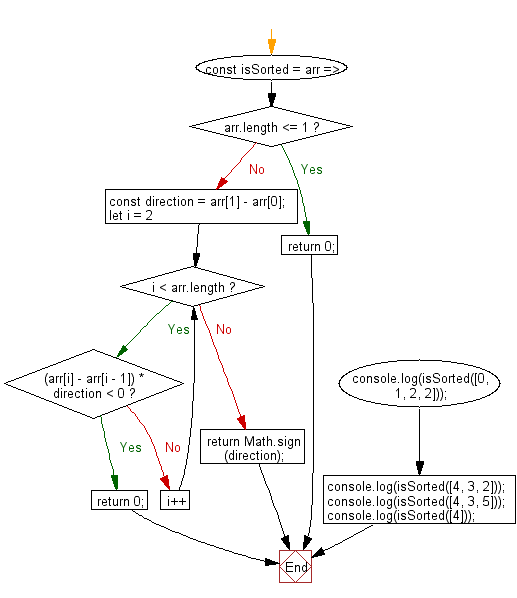# JavaScript Sorting Algorithm: Check if a numeric array is sorted or not

## JavaScript Sorting Algorithm: Exercise-24 with Solution

Write a JavaScript program to check if a numeric array is sorted or not.

• Loosely check if the array is sorted in descending order.
• Use Array.prototype.reverse() and Array.prototype.findIndex() to find the appropriate last index where the element should be inserted.

Sample Solution:

JavaScript Code:

``````const isSorted = arr => {
if (arr.length <= 1) return 0;
const direction = arr - arr;
for (let i = 2; i < arr.length; i++) {
if ((arr[i] - arr[i - 1]) * direction < 0) return 0;
}
return Math.sign(direction);
};
console.log(isSorted([0, 1, 2, 2]));
console.log(isSorted([4, 3, 2]));
console.log(isSorted([4, 3, 5]));
console.log(isSorted());
```
```

Sample Output:

```1
-1
0
0
```

Flowchart:Live Demo:

See the Pen javascript-common-editor by w3resource (@w3resource) on CodePen.

Improve this sample solution and post your code through Disqus

Previous: Sorted last index.
Next:Timsort sorting algorithm.

What is the difficulty level of this exercise?

Test your Programming skills with w3resource's quiz.

﻿